### Erin Conlisk, Michael Bloxham, John Conlisk, Brian Enquist, and John Harte. 2007. A new class of models of spatial distribution. Ecological Monographs 77:269–284.

Appendix D. Significance of results.

For the large samples here, performance rankings have high statistical significance. To illustrate, consider a test of the hypothesis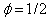, taking the bisection model as the maintained hypothesis. For each of the three data sets, Fig. 4 shows the log-likelihoodbelow the log-likelihood at the maximizing value of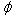, denote it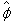. Is this difference significant?

A standard statistic for testing a simple hypothesis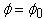against the composite alternative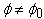is the likelihood ratio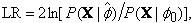(D.1)

where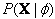denotes the likelihood function, with X the array of all data (here the collection of community landscapes). The ratio in brackets is by construction greater than or equal to one, hence LR is nonnegative. Large values of LR provide evidence against the hypothesis. If the hypothesisis true, LR is known to have approximately a chi-square distribution with one degree of freedom, assuming technical conditions. In addition to conditions that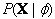be a well behaved function of, as it is here, a standard textbook condition is thatbe the product of likelihoods for a large number of independent draws from a single underlying distribution (Freund, 1962, Theorem 11.2). Here the likelihood functionis indeed a product of likelihoods for a large number of colonization assignments, left-right or up-down in the bisection model. However, assignments are not independent draws from a single distribution since the probability of left-vs-right or up-vs-down depends on previous assignments. Nonetheless, the chi-square result is often robust to departures from the standard textbook condition. To check robustness for the context here, modest Monte Carlo experiments were run (for relatively small dimension problems since computer speed is a severe limitation). For example, using a 16 × 16 grid, 500 landscapes N were generated for a single species with abundance n0 = 500, assuming the bisection model with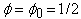. The value of LR was computed for each N, providing a histogram of 500 LR values. The 5%, 2.5%, and 1% cutoff values for the right tail of the histogram were 3.78, 5.12, and 6.32, in good agreement with exact chi-square cutoffs of 3.84, 5.02, and 6.64 (for one degree of freedom). These and related calculations give us confidence in the chi-square test for application here.

Continuing with the example, we wish to test the null hypothesisagainst the alternative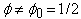under the bisection model. Consider the serpentine data set. The community log-likelihood is –7735 at the null value (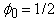) and –7440 at the maximizing value (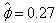). (These would be readable from Fig. 5 if it were precisely enough drawn; note that log-likelihoods on the graph are divided by 1000.) The resulting test statistic is LR = 2( –7440 + 7735) = 590, much above the 6.32 cutoff at a 1% significance level. To give an intuitive sense of these numbers, note from (D.1) that LR = 6.32 (the 1% value) implies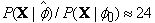. That is, significance at the 1% level requires the maximized probability of observing the data to be at least 24 times larger than the probability when. The serpentine value LR = 590 implies that the maximized probability is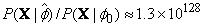more likely.

The corresponding LR values for the moist tropical and dry tropical data sets are still larger, LR = 10,660 and LR = 1476. Overall, it is vastly improbable, given the bisection model, that such large LR values would be observed ifwere true. Similar tests still more emphatically reject the random placement hypothesis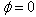in favor of the alternative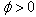.

In fact, because the data sets are large, it is typically quite easy to reject a particular valueusing an LR test based on the Fig. 5 log-likelihoods. To take an extreme example, consider an LR test of the hypothesis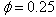against the alternative hypothesis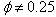. Sinceis close to the maximum likelihood values for the three data sets (,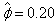,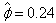), and since the log-likelihood curves are quite flat in the neighborhood of thesevalues, we might expect the LR test to accept the hypothesis. Yet the data sets are so large thatis rejected at a 5% level or better for all three data sets.

The LR statistic (D.1) is designed for testing a simple hypothesis against a composite alternative within the context of a single model – for example, testingagainst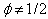for the bisection model. It is not appropriate for testing one simple hypothesis against another, within or across models. For example, it is not appropriate for either (i) testingvs.under the bisection model or (ii) testing the bisection model withagainst the quadrisection model with. In such cases, a log-likelihood statistic, such as 2ln[P(X | 0)/P(X | 1/2)] for example (i), may be appropriate; but the statistic will no longer have a chi-square distribution (it can be negative, for example). However, Monte Carlo experiments can be used to approximate the distributions of such test statistics, and tests can be based on the distributions. We have done modest Monte Carlo computations as a basis for a few such tests. As expected, they support the general claim that, for comparisons across models andvalues like those in the empirical section of the text, log-likelihood differences typically have high statistical significance.

An important caution is that mere statistical significance is not the main issue. With large data sets, very small differences may be statistically significant. The bigger question is the ecological importance of differences.

LITERATURE CITED

Freund, J. H. 1962. Mathematical statistics. Prentice-Hall, Englewood Cliffs, New Jersey, USA.

[Back to M077-008]